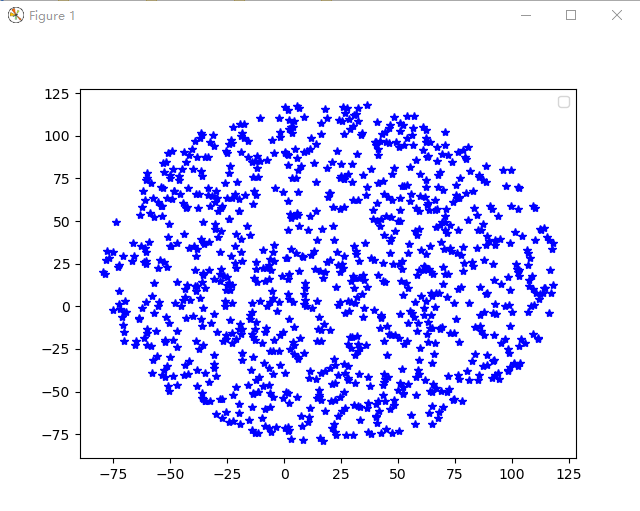• 2021-01-29 17:40:39

python在一个范围内取随机数的方法：

可以利用random.uniform()函数来实现。

random.uniform()函数用于生成一个指定范围内的随机浮点数，如：【random.uniform(10, 20)】。

random.randint(a, b)，用于生成一个指定范围内的整数。其中参数a是下限，参数b是上限，生成的随机数n: a <= n <= b。

代码示例：

print random.randint(12, 20)  # 生成的随机数 n: 12 <= n <= 20

print random.randint(20, 20)  # 结果永远是20

# print random.randint(20, 10)  # 该语句是错误的。下限必须小于上限

random.uniform(a, b)，用于生成一个指定范围内的随机符点数，两个参数其中一个是上限，一个是下限。如果a > b，则生成的随机数n: a >= n >= b。如果 a = n >= a。

代码示例：

print random.uniform(10, 20)

print random.uniform(20, 10)

# 18.7356606526

# 12.5798298022

内容扩展：

python3实现随机数

import random

#随机整数

import string

print(random.randint(0,99))

#随机选取0到100间的偶数

print(random.randrange(0, 101, 2))

#随机浮点数

print(random.random())

print(random.uniform(1, 10))

#随机字符

print(random.choice('abcdefg%^*f'))

#多个字符中选取特定数量的字符

print(random.sample('abcdefghij',3))

#多个字符中选取特定数量的字符组成新字符串

# print(string.join(random.sample(['a','b','c','d','e','f','g','h','i','j'], 3)).replace(" ",""))

#随机选取字符串

print(random.choice ( ['apple', 'pear', 'peach', 'orange', 'lemon'] ))

#洗牌

items = [1, 2, 3, 4, 5, 6]

random.shuffle(items)

print("洗牌:", items)

#从指定序列中随机获取k个元素作为一个片段返回，不会改变原有序列

list = []

list = random.sample(items,2)

print(list)

以上就是python在一个范围内取随机数的简单实例的详细内容，更多关于python如何在一个范围内取随机数的资料请关注猪先飞其它相关文章！

更多相关内容
• python在一个范围内取随机数的方法：可以利用random.uniform()函数来实现。random.uniform()函数用于生成一个指定范围内随机浮点数，如：【random.uniform(10, 20)】。random.randint(a, b)，用于生成一个指定范围...

python在一个范围内取随机数的方法：可以利用random.uniform()函数来实现。random.uniform()函数用于生成一个指定范围内的随机浮点数，如：【random.uniform(10, 20)】。random.randint(a, b)，用于生成一个指定范围内的整数。其中参数a是下限，参数b是上限，生成的随机数n: a <= n <= b。

代码示例：print random.randint(12, 20)  # 生成的随机数 n: 12 <= n <= 20

print random.randint(20, 20)  # 结果永远是20

# print random.randint(20, 10)  # 该语句是错误的。下限必须小于上限

random.uniform(a, b)，用于生成一个指定范围内的随机符点数，两个参数其中一个是上限，一个是下限。如果a > b，则生成的随机数n: a >= n >= b。如果 a = n >= a。

代码示例：print random.uniform(10, 20)

print random.uniform(20, 10)

# 18.7356606526

# 12.5798298022

展开全文• 在一个指定位置的园内随机取若干个坐标点，num为数量，radius为圆的半径，（centerx, centery）为圆心坐标。 import random import matplotlib.pyplot as plt def getRandomPointInCircle(num, radius, centerx, ...

方法一：根据条件判断的随机点产生方法

import random
import matplotlib.pyplot as plt

samplePoint = []
for i in range(num):
while True:
if (x ** 2) + (y ** 2) <= (radius ** 2):
samplePoint.append((int(x) + centerx, int(y) + centery))
break

plt.plot(x + centerx, y + centery, '*', color="blue")

return samplePoint

if __name__ == "__main__":
num = 1000
centerx,centery = 20, 20
samp = getRandomPointInCircle(num, radius, centerx, centery)
print("sample point" , samp)
plt.legend()
plt.show()


方法二：极坐标产生随机点

import random
import matplotlib.pyplot as plt

samplePoint = []
for i in range(num):
theta = random.random() * 2 * np.pi
r = random.uniform(0, radius ** 2)
x = math.cos(theta) * (r ** 0.5) + centerx
y = math.sin(theta) * (r ** 0.5) + centery
samplePoint.append((int(x), int(y)))
plt.plot(x, y, '*', color="blue")

return samplePoint

if __name__ == "__main__":
num = 1000
centerx,centery = 20, 20
samp = getRandomPointInCircle(num, radius, centerx, centery)
print("sample point" , samp)
plt.legend()
plt.show()


显示效果
显示如下：展开全文算法
•        js中，不同于python可以用random.sample(range(1,10),1)来获取任意指定范围的整数，只能获取[0,1)之间的小数，...这里，我们结合Math对象来求固定范围随机整数，...

在js中，不同于python可以用random.sample(range(1,10),1)来获取任意指定范围的整数，只能获取[0,1)之间的小数，因此需要我们再另行对它进行处理
在这里，我们结合Math对象来求取固定范围的随机整数，例如我想获得[m,n]之间的随机整数：

<script type="text/javascript">
function getrand(m,n){
var rand=Math.floor(Math.random()*(n-m+1))+m #核心代码
console.log(rand)
}
for(var i=1;i<=100;i++){
getrand(1,10);#取[1,10]
}
</script>


部分截图如下：展开全文随机数
• 如何使用MATLAB生成一个[a,b]范围内随机整数 比如：随机生成[9,13]范围内一个(或多个)整数~ 我搜索了网上很多博客，介绍的无外乎是： 要不就是0-x的，要不就是1-y范围的。 比如： randint() 这个函数高版本的...matlab rand 四舍五入取整
• a=randperm(80,1); 表示从1~80随机排列的整数中取出一个整数，同理 a=randperm(a,b); 表示从1~a随机排列的整数中取出b个整数
• Java 随机数之从指定数据范围内随机选取n不重复的数据 、简述 记--从指定数据范围内随机选取n不重复的数据，如从1~100中随机选取10数据。 二、效果 三、工程结构 四、源文件 import java.util...
• 1.如何生成一个数的二分之一的随机数,范围在正负0.3,如20的二分之你可以进入如图所示网站，右边那个框框中输入-30000,30000(不支持小数)，然后点击下面的按钮生成一个随机数，将它除以100000，就得到了你要的...
• Golang 伪随机/真随机取某个范围内的随机数 ???? Tue, 13 Nov 2018 by GolangNote golang 内置能实现伪随机(math/rand)和真随机(crypto/rand)的库。 真随机和伪随机概念 先大概了解一下伪随机和真随机的概念。根据...
• 方法： 7：30：00～8：00：00之间的随机时间： =RAND()*(VALUE(“8:00:00”)-VALUE(“7:30:00”))+VALUE(“7:30:00”) 下拉，将结果设为时间格式，显示为7:35:35。如要显示为07:35:35，需要自定义格式：hh:mm:ss。...
• 要取得[a,b)的随机整数，使用(rand() % (b-a))+ a; 要取得[a,b]的随机整数，使用(rand() % (b-a+1))+ a; 要取得(a,b]的随机整数，使用(rand() % ...要取得a到b之间的随机整数，另种表示：a + (int)b * rand() /...
• Java代码 //产生 一个范围 private int generateRandomByScope(int small,int bignum){ int num=-1; Random random=new Random(); num=random.nextInt(bignum)+small; //产生幸运 r...
• 这篇博客有详细方法 https://blog.csdn.net/u012759006/article/details/108252836python
• 该篇想展示的是我常用的种获取范围内随机整数的代码： 注意，展示的代码范围是 [ start , end ] ，也就基于这样已经满足我们的所有想实现的情况： /** * [0,10] */ int start=0; int end=10; int ...java 随机数
• python中用random模块来生成随机数。random模块的几种用法如下1.随机浮点数random() --- 产生大于等于0且小于1的浮点数ret = random.random()print(ret)uniform(a,b) --- ...随机整数randint(a,b) --- 产生a,b范围内...
•算法 java web spring ios
• Math.random()*305 的作用是0到305的随机数 加1 就是1到305的随机数, Math.floor是整数, 所以最后的结果是0到305的随机整数 微信小程序开发交流qq群 173683895 承接微信小程序开发。扫码加微信。 ...
• rand([m n])产生 m行，n列(0，1)范围内均匀分布的伪随机数 如果要求区间(a，b)由热心网友提供的答案1：实现方法：首先使用rand()函数生成N随机数，假设此时N随机数的和是S，那么将每随机数变为原rand([m n])...
• 50－100 randomize;  i:=random(50)+50;random
• 1、在j2se中使用Math.random()令系统随机选取一个0~1之间的double类型小数，将其乘以一个数，比如25，就能得到一个0~25范围内的随机数，这个在j2me中没有；  int randomNumber = (int) Math.round(Math.random...java
• 如何用MATLAB指定范围内生成多互不重叠的二维随机坐标 笔者做实验仿真时遇到了这问题，然后查阅了很多有关MATLAB生成不重叠的随机数的博客，但关于互不重叠的二维随机坐标的方法很少见，因此分享一下我的...MATLAB 二维坐标
• 除了代码，重要的是分析。。我实在想不明白这了，来求大神们帮帮忙java
• ## Hive表随机抽样取数

千次阅读 2020-06-18 21:53:20
1、按比例随机抽样 - 样本总量M, rand()<=(N/M+μ) 例如：如果抽10%的话 select * from table where rand()<=0.1;...特别注意的是：抽样迁先确认表的总记录 ...- 然后再这些随机样本里随机取N条 这样...hive 大数据
• 假设最大值为6，每次生成4个随机整数，生成随机数，生成随机数javascript
• 若要求为浮点数，则Python中只能近似达到这要求，因为随机函数的取值区间一般都为左闭右开区间，因为只能无限接近b。若要求为整数，那么将取数区间设置为[a,b+1)即可以到b了。具体如下：1. random()numpy.random...
• 产生区间[0,2]的一个随机数，结果可以为小数。 效果如下： 如果对你有帮助的话，给我点个赞，让我分享一些技术的时候更有动力。 另外我建了一个公众号，会不时分享前端的一些技术，或者遇到的难题和解决...Matlab 随机数
•  1、在j2se中使用Math.random()令系统随机选取一个0~1之间的double类型小数，将其乘以一个数，比如25，就能得到一个0~25范围内的随机数，这个在j2me中没有；  Java代码  int randomNumber = (int) Math.......